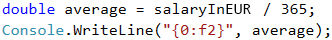# Problem: Daily Earnings

Ivan is a programmer in an American company, and he works at home approximately N days per month by earning approximately M dollars per day. At the end of the year, Ivan gets a bonus, which equals 2.5 of his monthly salaries. In addition, 25% of his annual salary goes for taxes.

Write a program that calculates what is the amount of Ivan's net average earnings in EUR per day, as he spends them in Europe. It is accepted that one year has exactly 365 days. The exchange rate of US dollar (USD) to Euro (EUR) will be read from the console.

## Input Data

Three numbers are read from the console.

• On the first line – work days per month. An integer within the range of [5 … 30].
• On the second line – daily earnings. A floating-point number within the range of [10.00 … 2000.00].
• On the third line – exchange rate of USD to EUR /1 dollar = X euro/. A floating-point number within the range of [0.05 … 4.99].

## Output Data

Print one number on the console – the daily earnings in EUR. The result will be rounded up to the second digit after the decimal point.

## Examples

Input Output
21
75.00
0.88
41.30

Explanation:

• One monthly salary = 21 * 75.00 = 1575 dollars.
• Annual income = 1575 * 12 month + 1575 * 2.5 bonus = 22837.5 dollars.
• Taxes = 25% of 22837.5 = 5709.375 dollars.
• Net annual income in USD = 22837.5 - 5709.375 = 17128.125 dollars.
• Net annual income in EUR = 17128.125 dollars * 0.88 = 15072.75 EUR.
• Average earnings per day = 15072.75 / 365 ≈ 41.30 EUR.
Input Output Input Output
15
105
0.875
41.06 22
199.99
0.892
116.93

## Hints and Guidelines

Firstly, we have to analyze the task and think of a way to solve it. Then, we will choose data types and, finally, we will write the code.

### Idea for Solution

Let's first calculate how much the monthly salary of Ivan is. We do that by multiplying the working days per month by his daily earnings. Firstly, we multiply the number by 12, so as to calculate his salary for 12 months, and then, we multiply it by 2.5 in order to calculate the bonus. After having summed up the two values, we calculate his annual income. Then, we reduce the annual income by 25% taxes. Depending on the exchange rate, we exchange the dollars (USD) to Euro (EUR) and after that we divide the result by 365 (days per year).

### Choosing Data Types

The working days per month are given as an integer, therefore, we can declare a variable of int type to store their value. For both the earned money and the exchange rate of USD to EUR, we will obtain a floating-point number, therefore, we will use double. As double is the data type with the higher scope, and the output should also be a floating-point number, we use double for the other variables that we create as well.

Again: after we have an idea on how to solve the problem and we have considered the data types that we are going to use, we can start writing the program. As in the previous tasks, we can divide the solution into three smaller tasks:

• Reading the input from the console.
• Doing the calculations.
• Printing the output on the console.

We declare the variables that we are going to use by trying to choose meaningful names. With Console.ReadLine(…) we read the input numbers from the console and we convert the input string to int or double with int/double.Parse(…).### Doing the Calculations

We do the calculations:We could write an expression that calculates the annual income without brackets as well. As multiplication is an operation that has a higher priority over addition, it will be performed first. Despite that, writing brackets is recommended when using more operators, as this way the code is easily readable and chances of making a mistake are smaller.

### Printing the Result

Finally, we have to print the result on the console. We notice that the number has to be rounded up to the second digit after the decimal point. In order to do that, we can use a placeholder – an item that will be replaced by a particular value when printing. In C#, a digit surrounded by curly brackets is used for a placeholder. As in programming counting starts from 0, the expression {0} means that it will be replaced by the first given argument. We can format an integer or a floating-point number by using F or f, which is followed by a whole positive number, specifying the number of digits after the decimal point: